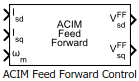Main Content

# ACIM Feed Forward Control

Decouple d-axis and q-axis current to eliminate disturbance

• Library:
• Motor Control Blockset / Controls / Control Reference

•## Description

The ACIM Feed Forward Control block decouples d-axis and q-axis current controls and generates the corresponding feed-forward voltage gains for field-oriented control of the induction motor.

The block accepts feedback values of d-axis and q-axis currents and the mechanical speed of the rotor.

### Equations

If you select `Per-Unit (PU)` in the Input units parameter, the block converts the inputs to SI units before performing any computation. After calculating the output, the block converts the values back to per-unit (PU) values.

The machine inductances and stator flux are represented as,

`$\sigma =1-\left(\frac{{L}_{m}^{2}}{{L}_{s}\cdot {L}_{r}}\right)$`

These equations describe how the block computes the feed-forward gain.

`${V}_{sd}^{FF}={\omega }_{e}{\lambda }_{sd}$`
`${V}_{sq}^{FF}=-{\omega }_{e}{\lambda }_{sq}$`

For detailed set of equations and assumptions, see Mathematical Model of Induction Motor.

where:

• ${\omega }_{e}$ is the electrical speed corresponding to frequency of stator voltages (Radians/ sec).

• ${L}_{ls}$ is the stator leakage inductance (Henry).

• ${L}_{lr}$ is the rotor leakage inductance (Henry).

• ${L}_{s}$ is the stator inductance (Henry).

• ${L}_{r}$ is the rotor inductance (Henry).

• ${L}_{m}$ is the magnetizing inductance of the motor (Henry).

• $\sigma$ is the total leakage factor of the induction motor.

• ${\lambda }_{sd}$ is the d-axis flux linkage of the stator (Weber).

• ${\lambda }_{sq}$ is the q-axis flux linkage of the stator (Weber).

• ${\lambda }_{rd}$ is the d-axis flux linkage of the rotor (Weber).

• ${i}_{sd}$ and ${i}_{sq}$ are the stator d- and q-axis currents (Amperes).

## Ports

### Input

expand all

Stator current along the d-axis of the rotating dq reference frame.

Data Types: `single` | `double` | `fixed point`

Stator current along the q-axis of the rotating dq reference frame.

Data Types: `single` | `double` | `fixed point`

Mechanical speed of the rotor.

Data Types: `single` | `double` | `fixed point`

### Output

expand all

Feed-forward voltage along the d-axis of the rotating dq reference frame.

Data Types: `single` | `double` | `fixed point`

Feed-forward voltage along the q-axis of the rotating dq reference frame.

Data Types: `single` | `double` | `fixed point`

## Parameters

expand all

Number of pole pairs available in the induction motor.

Inductance due to leakage flux linked to the stator winding (in Henry).

Inductance due to leakage flux linked to the rotor winding (in Henry).

Inductance due to the magnetizing flux (in Henry).

Rated flux of the induction motor (in Weber).

Saturation limit (in Volts) for the block outputs `VsdFF` and `VsqFF`.

Unit of the input values.

Base voltage (in Volts) for per-unit system.

#### Dependencies

To enable this parameter, set Input units to `Per-Unit (PU)`.

Base current (in Amperes) for per-unit system.

#### Dependencies

To enable this parameter, set Input units to `Per-Unit (PU)`.

Base speed (in rpm) for per-unit system.

#### Dependencies

To enable this parameter, set Input units to `Per-Unit (PU)`.

## See Also

### Topics

Introduced in R2020b

Get ebook